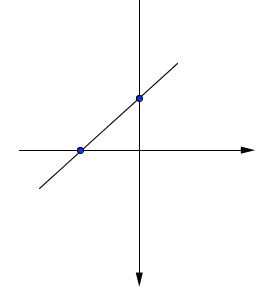### Home > GC > Chapter 1 > Lesson 1.1.5 > Problem1-43

1-43.

Using graph paper, draw a pair of $xy$-axes and scale them for $-6 \leq x \leq 6$ ($x$-values between and including $−6$ and $6$) and $−10 \leq y \leq 10$ ($y$-values between and including $−10$ and $10$). Complete the table below, substituting the $x$-values (inputs) to find the corresponding $y$-values (outputs) for the rule $y = x + 2$. Plot and connect the resulting points.

 x (input) y (output) $−4$ $−3$ $−2$ $−1$ $0$ $1$ $2$ 3 4

Line has a $y$-intercept of $(0, 2)$ and an $x$-intercept of $(− 2,0)$.

 x (input) y (output) $−4$ $−3$ $−2$ $−1$ $0$ $1$ $2$ 3 4 $-2$ $-1$ $0$ $1$ $2$ $3$ $4$ $5$ $6$